KR20150020953A - Method and apparatus for processing images - Google Patents

Method and apparatus for processing images Download PDF

Info

Publication number
KR20150020953A
KR20150020953A KR20130098134A KR20130098134A KR20150020953A KR 20150020953 A KR20150020953 A KR 20150020953A KR 20130098134 A KR20130098134 A KR 20130098134A KR 20130098134 A KR20130098134 A KR 20130098134A KR 20150020953 A KR20150020953 A KR 20150020953A
Authority
KR
South Korea
Prior art keywords
pixel
point
depth
set
depth value
Prior art date
Application number
KR20130098134A
Other languages
Korean (ko)
Inventor
최욱
이기창
정승원
Original Assignee
삼성전자주식회사
Priority date (The priority date is an assumption and is not a legal conclusion. Google has not performed a legal analysis and makes no representation as to the accuracy of the date listed.)
Filing date
Publication date
Application filed by 삼성전자주식회사 filed Critical 삼성전자주식회사
Priority to KR20130098134A priority Critical patent/KR20150020953A/en
Publication of KR20150020953A publication Critical patent/KR20150020953A/en

• 238000003709 image segmentation Methods 0 abstract 1
• 238000003672 processing method Methods 0 abstract 2

Images

•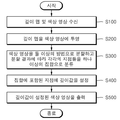•••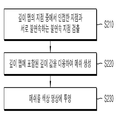•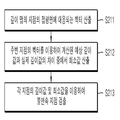••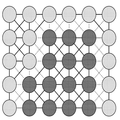••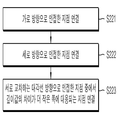•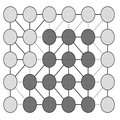•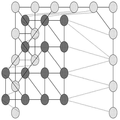•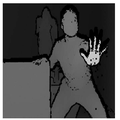•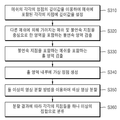•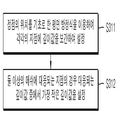••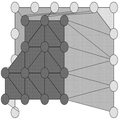•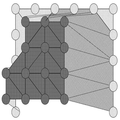•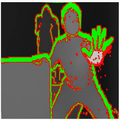•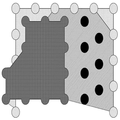•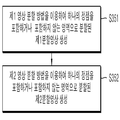•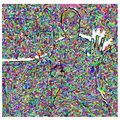•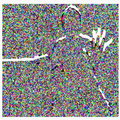•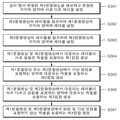••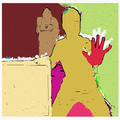•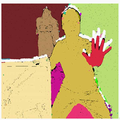•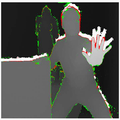•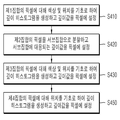•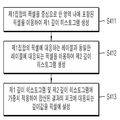•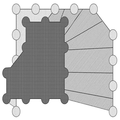•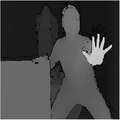•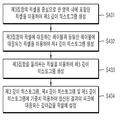•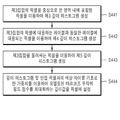••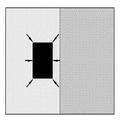•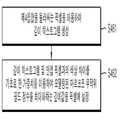••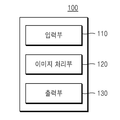Classifications

• GPHYSICS
• G06COMPUTING; CALCULATING; COUNTING
• G06KRECOGNITION OF DATA; PRESENTATION OF DATA; RECORD CARRIERS; HANDLING RECORD CARRIERS
• G06K9/00Methods or arrangements for reading or recognising printed or written characters or for recognising patterns, e.g. fingerprints
• G06K9/62Methods or arrangements for recognition using electronic means
• G06K9/6267Classification techniques
• GPHYSICS
• G06COMPUTING; CALCULATING; COUNTING
• G06TIMAGE DATA PROCESSING OR GENERATION, IN GENERAL
• G06T3/00Geometric image transformation in the plane of the image
• G06T3/40Scaling the whole image or part thereof
• G06T3/4053Super resolution, i.e. output image resolution higher than sensor resolution
• G06T3/4061Super resolution, i.e. output image resolution higher than sensor resolution by injecting details from a different spectral band
• GPHYSICS
• G06COMPUTING; CALCULATING; COUNTING
• G06TIMAGE DATA PROCESSING OR GENERATION, IN GENERAL
• G06T7/00Image analysis
• G06T7/10Segmentation; Edge detection
• G06T7/11Region-based segmentation
• GPHYSICS
• G06COMPUTING; CALCULATING; COUNTING
• G06TIMAGE DATA PROCESSING OR GENERATION, IN GENERAL
• G06T7/00Image analysis
• G06T7/50Depth or shape recovery
• GPHYSICS
• G06COMPUTING; CALCULATING; COUNTING
• G06TIMAGE DATA PROCESSING OR GENERATION, IN GENERAL
• G06T2200/00Indexing scheme for image data processing or generation, in general
• G06T2200/04Indexing scheme for image data processing or generation, in general involving 3D image data
• GPHYSICS
• G06COMPUTING; CALCULATING; COUNTING
• G06TIMAGE DATA PROCESSING OR GENERATION, IN GENERAL
• G06T2207/00Indexing scheme for image analysis or image enhancement
• G06T2207/10Image acquisition modality
• G06T2207/10028Range image; Depth image; 3D point clouds
• GPHYSICS
• G06COMPUTING; CALCULATING; COUNTING
• G06TIMAGE DATA PROCESSING OR GENERATION, IN GENERAL
• G06T2207/00Indexing scheme for image analysis or image enhancement
• G06T2207/20Special algorithmic details
• G06T2207/20212Image combination
• G06T2207/20221Image fusion; Image merging

Abstract

An embodiment of the present invention relates to an image processing method and an image processing method capable of upsampling a resolution of a depth map to a resolution of a color image using a color image having a resolution higher than a resolution of a depth map, A step of projecting each point included in the depth map onto a color image, a step of projecting the color map on which the depth map is projected using at least two or more image segmentation methods, Dividing the points included in the color image into at least one set according to each divided result, setting a depth value at a point of the color image included in the set, And outputting a color image in which a depth value is set.

Description

TECHNICAL FIELD  The present invention relates to an image processing method,

An embodiment of the present invention relates to an image processing method and an image processing apparatus, and more particularly, to an image processing method and an image processing apparatus that upsample a resolution of a depth map using a color image having a resolution higher than a resolution of a depth map, And more particularly, to an image processing method and an image processing method capable of performing image processing.

Traditionally, there have been a lot of researches on cameras that shoot 2D images, and many related products have been released. Conventionally, analog type cameras are mainly used, but in recent years, digital type cameras have been widely used. Research on digital cameras is also active. A study on a digital camera which can easily photograph a photograph having a high resolution and a high quality is continuing.

In recent years, along with the spread of the three-dimensional display device, the demand for the three-dimensional photographing device is increasing. Various types of 3D cameras are being sold, and technologies utilizing 3D cameras are also being developed.

For example, techniques have been introduced that can control an electronic device using a person's gesture without a physical controller such as a joystick. Also, thanks to the three-dimensional camera, it is much easier to create a three-dimensional model of a scene or object than in the prior art.

The three-dimensional camera has an advantage that it can provide three-dimensional position information of the surface of the object in real time. However, in general, a three-dimensional camera has a lower resolution than a two-dimensional camera. Among the 3D cameras commercialized so far, the resolution of the highest resolution product is 320 * 240 pixels. This is about 1/27 times the full HD resolution (1920 * 1080).

In addition, recent 3D cameras include not only a sensor for sensing depth but also a camera for detecting color. Thus, the high-resolution color information of the scene can be provided together with the three-dimensional depth information of the scene.

The embodiment of the present invention can provide an image processing method and an image processing method capable of upsampling the resolution of the depth map to the resolution of the color image using a color image having a higher resolution than the resolution of the depth map.

An embodiment of the present invention may provide an image processing method and an image processing method capable of upsampling the depth map such that the boundary in the depth map for the object matches the boundary in the color image for the object.

Embodiments of the present invention can provide an image processing method and an image processing method capable of upsampling a resolution of a depth map to a resolution higher than the resolution.

The embodiment of the present invention generates a depth map having the same viewpoint and resolution as the viewpoint and resolution of the color image using the depth map and the color image received through the depth sensor and the color camera, It is possible to provide an image processing method and an image processing apparatus that can be used.

The technical objects of the present invention are not limited to the above-mentioned technical problems, and other technical subjects not mentioned can be clearly understood by those skilled in the art from the following description.

The image processing method according to an embodiment of the present invention includes the steps of receiving a depth map and a color image for a specific scene, projecting each point included in the depth map onto the color image, And generating the divided result by dividing the projected color image by using at least two or more image segmentation methods and dividing the points included in the color image according to each divided result into at least one A step of classifying the color image into a set, a step of setting a depth value at a point of the color image included in the set, and a step of outputting the color image with the depth value set.

In addition, the resolution of the depth map may be lower than the resolution of the color image.

The step of projecting each of the points included in the depth map on the color image may further include using a depth value of each first point of the depth map and a depth value of a second point adjacent to the first point, Detecting a discontinuity point that is discontinuous with the second point in the first point, generating a mesh using the difference between the depth value of the first point and the depth value of the second point, And projecting the mesh onto the color image.

The step of detecting a discontinuity point that is discontinuous with the second point among the first points may include detecting a difference between a depth value of the first point and a depth value of the second point, Detecting the discontinuity using a determination result of whether or not the first object corresponding to the first point and the second object corresponding to the second point are the same among the first object and the second object.

The step of detecting a discontinuity point that is discontinuous with the second point among the first points may include calculating a vector corresponding to a tangent plane of the first point with respect to each first point of the depth map, An estimated depth value of the first point calculated by using the vector of at least one third point included in a specific range of areas centered on the first point with respect to each first point, Calculating a depth value of the first point, a depth value of the second point, the minimum value of the first point, and the minimum value of the second point, based on the depth value of the first point, And detecting a discontinuity point in the first point that is discontinuous with the second point.

The step of generating the mesh may further include connecting a fourth point adjacent to the first point to the first point and a fifth point adjacent to the first point in the longitudinal direction, And a step of connecting the fourth point to a depth of the fourth point and a depth value of the fifth point and a difference between a depth value of the fourth point and a depth value of the fifth point, And connecting the points corresponding to the smaller one of the difference between the depth value and the depth value of the first point to each other.

The step of classifying the points included in the color image into at least one or more sets may include the steps of setting a depth value at each point included in the projected mesh using the depth value of each vertex of the projected mesh Detecting at least one mesh including at least one vertex covered by another mesh and a discontinuity region including a specific range of areas centered at the discontinuity among the projected meshes, The method comprising: detecting, in a mesh, a hole region including at least one or more meshes including at least one discontinuity point, generating at least one virtual vertex in the hole region, And dividing the color image using the vertex and the virtual vertex It may phase, and according to the division result of each include the step of classifying said point included in the color image in the set.

The step of setting a depth value at each point included in the projected mesh may further include the step of calculating a depth value at each point included in the projected mesh using a plane equation based on the position of each vertex And setting a minimum depth value among the depth values corresponding to the points at a point corresponding to at least two or more meshes among the points included in the color image.

The step of dividing the color image using the at least two image segmentation methods, the vertices, and the virtual vertices may include one vertex or one virtual vertex using the first image segmentation method Generating a first divided image in which the color image projected from the mesh is divided into at least one region that does not include the vertex and the virtual vertex, and generating a second divided image, which is different from the first divided image, And generating a second divided image in which the color image is divided into at least one region including one vertex or one virtual vertex or not including the vertex and the virtual vertex, .

The step of classifying the points included in the color image into the set according to the respective divided results may include generating a third divided image in which the depth map is divided into at least one region, The method of claim 1, further comprising the steps of: setting a different label for each region included in the image; assigning a label corresponding to the label set in an area of the third divided image corresponding to the vertex included in each region included in the first divided image Corresponding to the label set in an area of the third divided image corresponding to the vertex included in each of the areas included in the second divided image, Setting a label in each region included in the second divided image, and selecting, from among the first pixels included in the first divided image, Wherein the label may include the step of generating a first set including the first pixels with each other and the label or more of the same at least one corresponding to a second pixel of the second image segment corresponding to the first pixel.

In addition, the step of setting a depth value at a point of the color image included in the set may include calculating a color of the third pixel and a position of the third pixel with respect to each third pixel included in the first set And a depth histogram corresponding to a peak of the depth histogram is set as a depth value of the third pixel.

In addition, the step of setting a depth value at a point of the color image included in the set may include, for each third pixel included in the first set, within a specific range area centered on the third pixel The depth value of at least one or more fourth pixels having the depth value set therein, the position difference between the third pixel and the fourth pixel, and the color difference between the third pixel and the fourth pixel, The depth value of the at least one fifth pixel corresponding to the same label as the label corresponding to the third pixel, the positional difference between the third pixel and the fifth pixel, Wherein the first depth histogram and the second depth histogram are weighted to produce a resultant peak pea k may be set as the depth value of the third pixel.

In addition, the step of classifying the points included in the color image into the set according to the respective divided results may further include a step of classifying the points corresponding to the first pixel or the region corresponding to the first pixel among the first pixels And generating a second set in which at least one of the regions corresponding to the second pixel includes at least one of the first pixels including the virtual vertices.

The step of setting a depth value at a point of the color image included in the set may include the step of setting a depth value at a point of the color image based on the color of the sixth pixel and the position of the sixth pixel, And the sixth pixel is divided into at least one subset including a seventh pixel set with the depth value, and the subset including the sixth pixel as the depth value of each sixth pixel, And a depth value of the seventh pixel is set.

In addition, the step of classifying the points included in the color image according to the divided result into the set may include: determining whether the label corresponding to the first pixel among the first pixels is included in the first pixel Wherein at least one of the region corresponding to the first pixel or the region corresponding to the second pixel corresponding to the first pixel is different from the label corresponding to the corresponding second pixel, And generating a third set of at least one of the first pixels.

In addition, the step of setting a depth value at a point of the color image included in the set may include, for each eighth pixel included in the third set, within a specific range area centered on the eighth pixel The depth value of at least one or more ninth pixels for which the depth value is set, the position difference between the eighth pixel and the ninth pixel, and the color difference between the eighth pixel and the ninth pixel, The depth value of the at least one tenth pixel corresponding to the same label as the label corresponding to the eighth pixel, the positional difference between the eighth pixel and the tenth pixel, A fourth depth histogram is generated using a color difference of 10 pixels, the depth value of at least one or more eleventh pixels surrounding the third set and the depth value is set, The fourth depth histogram, the fourth depth histogram, and the fourth depth histogram are generated using the difference in position between the eighth pixel and the eleventh pixel and the color difference between the eighth pixel and the eleventh pixel, 5 weighting is applied to the depth histogram to generate a summed sum histogram.

The depth value corresponding to a peak of the first sum histogram may be set as a depth value of the eighth pixel after the step of generating the sum histogram.

In addition, after the step of generating the sum histogram, a weight based on the color difference between the pixel adjacent to the eighth pixel and the eighth pixel, and a Markov random field model modeled using the summation histogram May be set as a depth value of the eighth pixel.

In addition, the step of classifying the points included in the color image into the set according to the respective divided results may further include, in each of the first pixels, the region corresponding to the first pixel, And generating a fourth set in which the region corresponding to the corresponding second pixel includes at least one or more of the first pixels that do not include the vertex and the virtual vertex.

In addition, the step of setting a depth value at a point of the color image included in the set may include, for each of the twelfth pixels included in the fourth set, at least one or more pixels surrounding the fourth set, And generating the depth histogram using the depth value of the thirteenth pixel and the positional difference between the twelfth pixel and the thirteenth pixel.

The depth value corresponding to a peak of the depth histogram may be set as a depth value of the twelfth pixel after the step of generating the depth histogram.

In addition, after the step of generating the depth histogram, a weight based on a color difference between the pixel adjacent to the twelfth pixel and the twelfth pixel, and a Markov Random Field score modeled using the depth histogram May be set as a depth value of the twelfth pixel.

An image processing apparatus according to an embodiment of the present invention includes an input unit for receiving or generating a depth map or a color image for a specific scene, a projection unit for projecting each point included in the depth map on the color image, A method of generating a color image in which a map is projected, the method comprising the steps of: generating each divided result using at least two or more image segmentation methods; classifying points included in the color image into at least one set according to each divided result; An image processing unit for setting a depth value at a point of the color image included in the set, and an output unit for outputting the color image with the depth value set.

According to an embodiment of the present invention, the resolution of the depth map can be upsampled to the resolution of the color image using a color image having a higher resolution than the resolution of the depth map.

According to an embodiment of the present invention, the depth map can be upsampled such that the boundary in the depth map for the object coincides with the boundary in the color image for the object.

According to the embodiment of the present invention, the resolution of the depth map can be upsampled to a resolution higher than the resolution.

According to the embodiment of the present invention, a depth map having the same viewpoint and resolution as the viewpoint and resolution of the color image using the depth map and the color image respectively received through the depth sensor and the color camera, Can be generated.

FIG. 1 is a flowchart illustrating a procedure in which an image processing method according to an exemplary embodiment of the present invention is performed.
2 is a graphical representation of a received depth map according to an embodiment of the present invention.
3 is a diagram illustrating a received color image according to an embodiment of the present invention.
FIG. 4 is a flowchart illustrating a procedure in which a depth map is projected onto a color image according to an embodiment of the present invention.
FIG. 5 is a flowchart showing a sequence of detecting discontinuous points that are discontinuous with adjacent points among the points of the depth map according to the embodiment of the present invention.
FIG. 6 is a reference diagram for explaining a process of calculating a predicted depth value at a specific point using a vector of a surrounding point according to an embodiment of the present invention.
FIG. 7 is a conceptual diagram showing a result of detecting discontinuous points that are discontinuous with adjacent points among the points of the depth map according to the embodiment of the present invention. FIG.
FIG. 8 is a diagram showing a result of detecting discontinuous points that are discontinuous with adjacent points among the points of the depth map according to the embodiment of the present invention. FIG.
FIG. 9 is a flowchart illustrating a sequence in which meshes are generated according to an embodiment of the present invention.
10 is a conceptual diagram illustrating a result of generating a mesh according to an embodiment of the present invention.
11 is a conceptual diagram illustrating a result of projection of a mesh onto a color image according to an embodiment of the present invention.
12 is a diagram showing a result of a projection of a mesh onto a color image according to an embodiment of the present invention.
13 is a flowchart illustrating a procedure in which a color image is divided into two or more methods according to an embodiment of the present invention, and each point is classified into one or more sets according to the division result.
FIG. 14 is a flowchart illustrating a procedure of setting depth values at respective points included in a mesh using depth values of respective vertices of a mesh according to an embodiment of the present invention. FIG.
15 is a conceptual diagram illustrating a result of setting depth values at respective points included in a mesh according to an embodiment of the present invention.
16 is a conceptual diagram showing a result of detecting a discontinuous area according to an embodiment of the present invention.
17 is a conceptual diagram showing a result of detection of a hole region according to an embodiment of the present invention.
18 is a diagram showing a result of detecting a discontinuous area and a hole area according to an embodiment of the present invention.
19 is a conceptual diagram showing a result of generating a virtual vertex in a hole region according to an embodiment of the present invention.
FIG. 20 is a flowchart illustrating a procedure of dividing a color image using two or more image segmentation methods according to an embodiment of the present invention.
FIG. 21 is a diagram showing a result of dividing a color image using a first image segmentation method according to an embodiment of the present invention.
22 is a diagram showing a result of dividing a color image using a second image segmentation method according to an embodiment of the present invention.
23 is a flowchart showing a procedure of sorting each point of a color image into one or more sets according to an embodiment of the present invention.
FIG. 24 is a diagram showing a divided image obtained by dividing a depth map according to an embodiment of the present invention. FIG.
FIG. 25 is a diagram visually showing a result of setting a label in each divided area using the first image segmentation method according to an embodiment of the present invention. FIG.
FIG. 26 is a diagram showing a result of setting a label in each of divided areas using the second image segmentation method according to an embodiment of the present invention. FIG.
FIG. 27 is a diagram showing a result of visualizing each of the points of a color image classified into one or more sets according to an embodiment of the present invention.
28 is a flowchart showing a procedure of setting a depth value at a point included in the set according to an embodiment of the present invention.
29 is a flowchart showing a sequence in which a depth histogram is generated for a first set of pixels and depth values are set in pixels according to an embodiment of the present invention.
30 is a conceptual diagram illustrating a result of dividing a second set of pixels into subsets according to an embodiment of the present invention.
31 is a diagram showing a result of visually showing a result of setting a depth value in a second set of pixels according to an embodiment of the present invention.
32 is a flowchart showing a sequence in which a depth histogram is generated for a third set of pixels and depth values are set in pixels according to an embodiment of the present invention.
33 is a flowchart illustrating a procedure in which a depth histogram is generated for a third set of pixels and a depth value is set for pixels according to another embodiment of the present invention.
FIG. 34 is a flowchart showing a sequence in which a depth histogram is generated for a fourth set of pixels and depth values are set in pixels according to an embodiment of the present invention. FIG.
35 is a reference diagram for explaining a process of generating a depth histogram using pixels surrounding a fourth set according to an embodiment of the present invention.
FIG. 36 is a flowchart showing a sequence in which a depth histogram is generated for a fourth set of pixels and depth values are set in pixels according to another embodiment of the present invention. FIG.
FIG. 37 is a diagram showing a result of outputting a color image in which a depth value is set according to an embodiment of the present invention.
38 is a block diagram showing a configuration of an image processing apparatus according to an embodiment of the present invention.

BRIEF DESCRIPTION OF THE DRAWINGS The advantages and features of the present invention and the manner of achieving them will become apparent with reference to the embodiments described in detail below with reference to the accompanying drawings. The present invention may, however, be embodied in many different forms and should not be construed as being limited to the embodiments set forth herein. Rather, these embodiments are provided so that this disclosure will be thorough and complete, and will fully convey the scope of the invention to those skilled in the art. Is provided to fully convey the scope of the invention to those skilled in the art, and the invention is only defined by the scope of the claims. Like reference numerals refer to like elements throughout the specification.

Although "first" or "second" and the like are used to describe various components, such components are not limited by such terms. Such terms may be used to distinguish one element from another. Therefore, the first component mentioned below may be the second component within the technical spirit of the present invention.

The terminology used herein is for the purpose of illustrating embodiments and is not intended to be limiting of the invention. In the present specification, the singular form includes plural forms unless otherwise specified in the specification. &Quot; comprises "or" comprising "as used herein mean that the stated element or step does not exclude the presence or addition of one or more other elements or steps.

Unless defined otherwise, all terms used herein are to be construed in a sense that is commonly understood by one of ordinary skill in the art to which this invention belongs. In addition, commonly used predefined terms are not ideally or excessively interpreted unless explicitly defined otherwise.

Hereinafter, an image processing method and an image processing apparatus 100 according to an embodiment of the present invention will be described in detail with reference to FIG. 1 to FIG.

FIG. 1 is a flowchart illustrating a procedure in which an image processing method according to an exemplary embodiment of the present invention is performed. Referring to FIG. 1, an image processing method according to an exemplary embodiment of the present invention may include performing a step S100 of receiving a depth map and a color image for a specific scene.

2 is a graphical representation of a received depth map according to an embodiment of the present invention. The depth map can be generated by a depth sensor. Also, the depth map may be the result of transforming the sensed result by the depth sensor. The depth sensor can be, for example, any of a stereoscopic camera, a pattern projection camera, a TOF (Time Of Flight) camera, or a similar measuring device.

3 is a diagram illustrating a received color image according to an embodiment of the present invention. The color image can be generated by the optical photographing apparatus. In addition, the color image may be the result of conversion of the result of photographing by the optical photographing apparatus. The optical photographing apparatus may be, for example, a CMOS camera, a CCD camera (Charge-Coupled Device camera), or a similar photographing apparatus.

According to the embodiment of the present invention, the depth map and the color image can be respectively received through the depth sensor and the color camera having the same position and direction of the viewpoint. Also, the resolution of the color image may be higher than the resolution of the depth map.

Referring again to FIG. 1, a step S200 of projecting each point included in the depth map on the color image may be performed. FIG. 4 is a flowchart illustrating a procedure in which a depth map is projected onto a color image according to an embodiment of the present invention.

Referring to FIG. 4, in step S200, a depth map is projected onto a color image using a depth value of each first point of the depth map and a depth value of a second point adjacent to the first point, , A step S210 of detecting a discontinuous point that is discontinuous with the second point among the first points may be performed. FIG. 5 is a flowchart showing a sequence of detecting discontinuous points that are discontinuous with adjacent points among the points of the depth map according to the embodiment of the present invention.

Referring to FIG. 5, a step S210 of detecting a discontinuity point that is discontinuous with an adjacent point among the points of the depth map is performed by first obtaining, for each first point of the depth map, a vector corresponding to a tangent plane of the first point (S211) may be performed.

According to an embodiment of the present invention, it is possible to determine whether discontinuity between first points adjacent to each other in the depth map is determined using a predetermined threshold value. If it is assumed that the normal direction of the continuous surface of the object and the viewing direction of the depth sensor are at an angle greater than a certain reference angle, then it may be greater than the threshold value of the depth value of each of the adjacent first points . Thus, in practice, it can be determined that the surface of the object has discontinuity even though it has continuity. Therefore, as in the embodiment of the present invention, when a vector corresponding to the tangential plane of the first fingernail is calculated and used, the above-mentioned error can be prevented because the element for the normal direction of the surface of the object is considered.

According to the embodiment of the present invention, a vector corresponding to the tangential plane of the first point can be calculated using the depth value of each point included in the 3 * 3 region centered on any one first point have. For example, the depth value du of the first point u can be expressed by the following equation (1).

Therefore, according to the embodiment of the present invention, by using the position vector (x, y) of each point included in the 3 * 3 region and the depth value of each point,

Can be calculated. If the above process is repeated for every point included in the depth map, the vector may be calculated for each point.

Next, an estimated depth value of the first point calculated using the vector of at least one third point included in a specific range of the area about the first point with respect to each of the first points, The step S212 of calculating the minimum value among the differences of the depth values of the first point included in the depth map may be performed. FIG. 6 is a reference diagram for explaining a process of calculating a predicted depth value at a specific point using a vector of a surrounding point according to an embodiment of the present invention.

As shown in FIG. 6, the predicted depth value of the first point can be calculated using the previously calculated vector for each point included in the area of the 3 * 3 size centered on the first point. In other words, eight predicted depth values for the first point can be calculated using each of the eight third points except for the first point among the points included in the area. The expected depth value can be calculated by discarding the vector corresponding to the position of the first point and the previously calculated vector for the third point.

The difference between the calculated expected depth value and the actual depth value of the first point included in the depth map can be calculated. Referring to FIG. 6, since a total of 8 estimated depth values can be calculated, a total of 8 difference values can be calculated. Also, the minimum value among the calculated difference values may be calculated. If the above process is repeated for all points included in the depth map, the minimum value may be calculated for each point.

According to the above description, the minimum value lu corresponding to the first point u can be expressed by the following equation (2).

In Equation (2), Nu is a set including a third point u 'included in a region of a specific size centered on the first point u. In Equation (2), u is a position vector of the first point u. In Equation (2), du is the depth value of the first point u included in the depth map. Also, lu 'is a vector corresponding to the tangent plane of the third point u'.

Next, using the depth value of the first point, the depth value of the second point, the minimum value of the first point and the minimum value of the second point, A step S213 of detecting a discontinuity point may be performed.

For example, the smaller the difference between the depth value of the first point and the depth value of the second point, the higher the probability that the first point and the second point have continuity. Therefore, the discontinuity can be determined by comparing the difference between the depth value of the first point and the depth value of the second point with the threshold value.

The discontinuity may be determined according to a determination result as to whether or not a first object corresponding to the first point and a second object corresponding to the second point are the same among at least one object existing in the scene . The determination as to whether the first object and the second object are equal to each other can be performed as follows.

The smaller the difference between the estimated depth value of the first point and the actual depth value of the first point included in the depth map calculated using the vector corresponding to the tangent plane of the second point, the higher the probability of having continuity . The smaller the difference between the estimated depth value of the second point calculated using the vector corresponding to the tangent plane of the first point and the actual depth value of the second point included in the depth map, Can be high.

Therefore, the discontinuity can be determined by comparing the difference value with the threshold value. The predicted depth value of the first point or the second point is calculated by deducing a vector corresponding to the position of the first point or the second point and a minimum value calculated previously for the second point or the first point .

According to the above description, a discontinuity index e as shown in the following equation (3) can be calculated to determine whether there is discontinuity between the first point u and the second point u 'adjacent to the first point u. Also, when the discontinuity index e is compared with a predefined threshold value, if the discontinuity index e is greater than or equal to the threshold value, the first point u and the second point u 'may be determined to have discontinuities.

In Equation (3), du is the depth value of the first point u and du 'is the depth value of the second point u'. The vector u is the position vector of the first point u and the vector u 'is the position vector of the second point u'. The vector lu 'is the minimum value calculated previously for the second point u', and the vector lu is the minimum value calculated for the first point u.

FIG. 7 is a conceptual diagram showing a result of detecting discontinuous points that are discontinuous with adjacent points among the points of the depth map according to the embodiment of the present invention. FIG. Referring to FIG. 7, when two neighboring points have discontinuities, an edge connecting the two points is represented by a dotted line. In addition, when two adjacent points have continuity, the trunk connecting the two points is represented by a solid line. Referring to FIG. 7, a portion constituted by black points may have a discontinuity with a portion constituted by gray points. Therefore, the portion composed of black points and the portion composed of gray points may be different objects.

FIG. 8 is a diagram showing a result of detecting discontinuous points that are discontinuous with adjacent points among the points of the depth map according to the embodiment of the present invention. FIG. Referring to FIG. 8, a set of discontinuous points may represent a boundary of an object included in a scene.

Referring again to FIG. 4, a difference between the depth value of the first point and the depth value of the second point, and generating the mesh using the first point may be performed (S220). FIG. 9 is a flowchart illustrating a sequence in which meshes are generated according to an embodiment of the present invention.

Referring to FIG. 9, a step S220 of creating a mesh may be performed by connecting a first point and a fourth point adjacent to each other in the horizontal direction (S221) with the first point. Next, connecting the first point and the fifth point adjacent to each other in the longitudinal direction with the first point (S222) may be performed.

Next, the difference between the depth value of the fourth point and the depth value of the fifth point and the depth value of the sixth point adjacent to the fourth point in the longitudinal direction and adjacent to the fifth point in the width direction, The step of connecting the points corresponding to the smaller one among the differences of the depth values of the one point (S223) may be performed. In other words, the four points that make up the rectangle can be divided into two in the diagonal direction. Among the two pairs of points, the pair of smaller difference in depth value corresponding to the point may be connected to each other and the other pair may not be connected. 10 is a conceptual diagram illustrating a result of generating a mesh according to an embodiment of the present invention.

Referring again to FIG. 4, the step of projecting the mesh onto the color image (S230) may be performed. The depth sensor and the color camera may be calibrated to each other in advance. In other words, mapping information on which point of the depth map detected by the depth sensor corresponds to which point of the color image photographed by the color camera may be stored in advance. Accordingly, the mesh can be projected onto the color image by referring to the mapping information.

11 is a conceptual diagram illustrating a result of projection of a mesh onto a color image according to an embodiment of the present invention. As shown in the left part of FIG. 11, some vertices of the mesh may overlap each other due to the difference in viewpoint between the depth sensor and the color camera. On the contrary, as shown in the right part of FIG. 11, there may be a part where the density of the vertex is lower than other parts. 12 is a diagram showing a result of a projection of a mesh onto a color image according to an embodiment of the present invention.

Referring again to FIG. 1, the color image, in which the depth map is projected, is generated by using at least two or more image segmentation methods, (S300) of classifying the included points into at least one set may be performed. 13 is a flowchart illustrating a procedure in which a color image is divided into two or more methods according to an embodiment of the present invention, and each point is classified into one or more sets according to the division result.

Referring to FIG. 13, in step S300, points included in a color image are classified into one or more sets. First, a depth value of each vertex of the projected mesh is used to determine the position of each point included in the projected mesh A step S310 of setting a depth value may be performed. FIG. 14 is a flowchart illustrating a procedure of setting depth values at respective points included in a mesh using depth values of respective vertices of a mesh according to an embodiment of the present invention. FIG.

Referring to FIG. 14, the step of setting a depth value at each point included in the mesh (S310) may be performed by first calculating a depth value of each point included in the projected mesh using a plane equation based on the position of each vertex And interpolating and setting the depth value at a point (S311) may be performed. Each mesh can be in the shape of a triangle. Therefore, a plane equation can be calculated based on the position and depth values of three vertices included in the mesh. The depth values may be set at points in each of the meshes using the planar equation.

Next, a minimum depth value among the depth values corresponding to the points may be set at a point corresponding to at least two of the points included in the color image (S312). For example, some points may overlap at least two or more meshes, such as the points included in the left portion of FIG. In this case, according to the conventional Z-buffering method, the smallest depth value among the corresponding depth values can be set at the above point. In other words, the mesh may be obscured at least partially by other meshes.

15 is a conceptual diagram illustrating a result of setting depth values at respective points included in a mesh according to an embodiment of the present invention. Referring to FIG. 15, some of the projected meshes in FIG. 11 are partially obscured by other meshes by the Z-buffering method. Referring to FIG. 15, the depth value corresponding to the mesh of the black portion may be smaller than the depth value corresponding to the gray portion mesh.

Referring again to FIG. 13, in the projected mesh, at least one mesh including at least one vertex covered by another mesh and a discontinuity region including a specific range of the center around the discontinuity point are detected Step S320 may be performed. If at least one of the three vertexes included in the mesh is covered by another mesh, the area inside the mesh may be determined as a discontinuous area. In addition, the region of the specific range centered on the discontinuity point detected in the step S210 of detecting the discontinuity point may be determined as a discontinuous region.

16 is a conceptual diagram showing a result of detecting a discontinuous area according to an embodiment of the present invention. Referring to FIG. 16, a grid pattern is filled in a region detected as a discontinuous region.

Referring again to FIG. 13, a step S330 of detecting a hole area including at least one mesh including at least one discontinuity point among the projected meshes may be performed. 17 is a conceptual diagram showing a result of detection of a hole region according to an embodiment of the present invention. Referring to FIG. 17, a grid pattern is filled in a region detected as a discontinuous area. 18 is a diagram showing a result of detecting a discontinuous area and a hole area according to an embodiment of the present invention. In Fig. 18, the area indicated by red corresponds to the discontinuous area, and the area indicated with green corresponds to the hole area.

Referring again to FIG. 13, a step S340 of creating at least one virtual vertex in the hole region may be performed. 19 is a conceptual diagram showing a result of generating a virtual vertex in a hole region according to an embodiment of the present invention. Referring to FIG. 19, a black virtual vertex is shown on the right side, which is a hole area.

Referring again to FIG. 13, a step S350 of dividing the color image on which the depth map is projected using two or more image segmentation methods may be performed. It can be seen that any one kind of image segmentation method does not always show better performance than all other image segmentation methods. According to the embodiment of the present invention, the boundary of an object at a high resolution can be more accurately detected by using at least two image segmentation methods.

FIG. 20 is a flowchart illustrating a procedure of dividing a color image using two or more image segmentation methods according to an embodiment of the present invention. Referring to FIG. 20, in step S350, the color image is divided into a plurality of vertices or a plurality of virtual vertices by using a first image segmentation method, (S351) may be performed to generate a first divided image in which the color image projected from the mesh is divided into at least one or more regions. In other words, one divided area may contain only one vertex or virtual vertex, or may not contain either a correction or a virtual vertex.

For example, the first image segmentation method may be an SLIC image segmentation method. According to the method, all the points included in the color image can be divided so as to be included in the same area as the vertex closest to the point. In this case, the distance from the point to the apex may be a result of squaring each of the difference in color and position of the point and the apex, adding weights, and summing the weights.

At this time, there may be a case where the first point and the vertex can not be physically connected to each other because there is a second point between the vertex closest to the first point and the second vertex included in another region including the vertex have. In this case, the first point may be defined as belonging to an area that does not include either a vertex or a virtual vertex. FIG. 21 is a diagram showing a result of dividing a color image using a first image segmentation method according to an embodiment of the present invention.

Referring to FIG. 20 again, at least one vertex or one virtual vertex is included or a plurality of virtual vertices including no vertex and the virtual vertex are generated using a second image segmentation method different from the first image segmentation method A step S352 of generating a second divided image in which the color image projected from the mesh is divided into one or more regions may be performed.

The second image segmentation method may be an EGB image segmentation method. According to the above method, when the color difference is smaller than the threshold value for all adjacent points, they can be connected to each other and included in the same area. The threshold value may be inversely proportional to the size of the area connected to each other. In other words, the larger the area, the smaller the threshold value, so the possibility that a new point can be included in the same area can be lowered.

If one of the two points is a vertex, or one of the two points belongs to an area containing a vertex, the label of the vertex may be replaced with the label of the generated area. If both points are vertices, or if they belong to an area containing vertices, it is not necessary to connect the two points. 22 is a diagram showing a result of dividing a color image using a second image segmentation method according to an embodiment of the present invention.

Referring again to FIG. 13, classification of each point into one or more sets according to the division result (S360) may be performed. 23 is a flowchart showing a procedure of sorting each point of a color image into one or more sets according to an embodiment of the present invention.

Referring to FIG. 23, in step S360 of classifying each point into one or more sets according to the division result, a third divided image in which the depth map is divided into at least one region is generated, A step S361 of setting different labels in the respective regions included in the divided images may be performed.

The depth map may be image segmentation using, for example, a Watershed or EGB image segmentation method. Also, different labels may be set for each divided area. FIG. 24 is a diagram showing a divided image obtained by dividing a depth map according to an embodiment of the present invention. FIG. Referring to FIG. 24, areas having different labels are shown in different colors.

Referring again to FIG. 23, a label corresponding to the label set in the region of the third divided image corresponding to the vertex included in each of the regions included in the first divided image is referred to as the first divided image (S362) may be performed.

FIG. 25 is a diagram visually showing a result of setting a label in each divided area using the first image segmentation method according to an embodiment of the present invention. FIG. Referring to Fig. 25, regions having different labels are shown in different colors. Referring to FIG. 25, it is confirmed that a large number of areas in FIG. 21 are merged with each other.

Referring again to FIG. 23, a label corresponding to the label set in the region of the third divided image corresponding to the vertex included in each region included in the second divided image is referred to as the second divided image (Step S363) may be performed.

FIG. 26 is a diagram showing a result of setting a label in each of divided areas using the second image segmentation method according to an embodiment of the present invention. FIG. Referring to FIG. 26, regions having different labels are shown in different colors. Referring to FIG. 26, it is confirmed that a large number of areas in FIG. 22 are merged with each other.

Referring again to FIG. 23, among the first pixels included in the first divided image, the label corresponding to the first pixel corresponds to the second pixel of the second divided image corresponding to the first pixel (S364) may be performed to generate a first set including at least one or more first pixels that are the same as the label.

At least one of the regions corresponding to the first pixels or the regions corresponding to the second pixels corresponding to the first pixels among the first pixels includes at least one of the regions including the virtual vertices, A step S365 of generating a second set including the first pixel may be performed. In other words, the second set may include the pixels contained in the hole area.

Next, among the first pixels, the label corresponding to the first pixel is different from the label corresponding to the second pixel corresponding to the first pixel, and the label corresponding to the first pixel (S366) is performed to generate a third set including at least one or more of the first pixels including at least one of the regions corresponding to the first pixels or the regions corresponding to the second pixels corresponding to the first pixels . In other words, the third set may include pixels having different results based on the first image segmentation scheme and the second image segmentation scheme.

Next, among the first pixels, the region corresponding to the first pixel and the region corresponding to the second pixel corresponding to the first pixel do not include the vertex and the virtual vertex, and at least one The generating of the fourth set including the first pixel (S367) may be performed. In other words, the fourth set may include pixels that do not belong to a region including a vertex or a virtual vertex.

As described above, between the arbitrary first point and the closest vertex, there is a second point included in the region different from the region including the vertex, so that the first point and the vertex can not be physically connected to each other There may be cases. In this case, the first point may be defined as belonging to an area that does not include either a vertex or a virtual vertex. And the fourth set may include the first point.

FIG. 27 is a diagram showing a result of visualizing each of the points of a color image classified into one or more sets according to an embodiment of the present invention. Referring to FIG. 27, the pixels included in the first set are shown in gray, and the pixels included in the second set are shown in white. In addition, the pixels included in the third set are shown in green, and the pixels included in the fourth set are shown in red.

Referring again to FIG. 1, setting a depth value at a point of the color image included in the set (S400) may be performed. 28 is a flowchart showing a procedure of setting a depth value at a point included in the set according to an embodiment of the present invention.

Referring to FIG. 28, in step S400, a depth value is set at a point of the color image included in the set. First, a depth histogram is generated based on hue and position for a first set of pixels, (S410) may be performed.

29 is a flowchart showing a sequence in which a depth histogram is generated for a first set of pixels and depth values are set in pixels according to an embodiment of the present invention. Referring to FIG. 29, a first depth histogram may be generated for each third pixel included in the first set (S411).

Wherein the first depth histogram includes the depth value of at least one or more fourth pixels included in a region of a specific range centered on the third pixel and in which the depth value is set, And a color difference between the third pixel and the fourth pixel. For example, the first depth histogram may be generated using the following equation (4).

In Equation (4), G is a Gaussian weight function. Ix represents the color of the pixel x. x 'represents a pixel in which a depth value is set among pixels included in a specific range of the area W (x) centered at x. d 'represents the depth value of the pixel for which the depth value is set.

Next, the depth value of at least one fifth pixel corresponding to the same label as the label corresponding to the third pixel, the positional difference between the third pixel and the fifth pixel, and the difference between the third pixel and the fifth pixel The step of generating the second depth histogram using the color difference of the pixel (S412) may be performed. For example, the second depth histogram can be generated using the following equation (5).

In Equation (5), R (x) is a set of pixels divided by the above-described image segmentation method and included in an area including the pixel x.

Next, a step S413 of setting the depth value corresponding to a peak of a result obtained by weighting the first depth histogram and the second depth histogram as a depth value of the third pixel is performed . The result obtained by applying the weights to the first depth histogram and the second depth histogram may be expressed as Equation (6).

Referring again to FIG. 28, next, each sixth pixel included in the second set is selected based on the color of the sixth pixel and the position of the sixth pixel, and the depth value is set to one A depth value of the seventh pixel included in the subset including the sixth pixel is set as a depth value of each sixth pixel, the depth value being divided into at least one subset including a seventh pixel S420) may be performed.

30 is a conceptual diagram illustrating a result of dividing a second set of pixels into subsets according to an embodiment of the present invention. Depth values are set for some pixels around the hole area. Accordingly, the second set can be divided into sub-sets using the Watershed algorithm or the EGB image segmentation method or the like using the pixels as vertices. Thereby, all the pixels included in the hole area can be included in the same area as one vertex. Also, the depth value of the new vertex may be set as the depth value of the pixels included in the subset. 31 is a diagram showing a result of visually showing a result of setting a depth value in a second set of pixels according to an embodiment of the present invention.

Referring again to FIG. 28, a depth histogram may be generated based on hue and position for a third set of pixels, and a step of setting a depth value to a pixel (S430) may be performed. 32 is a flowchart showing a sequence in which a depth histogram is generated for a third set of pixels and depth values are set in pixels according to an embodiment of the present invention.

Referring to FIG. 32, for each eighth pixel included in the third set, at least one or more ninth pixels included in a specific range region centered on the eighth pixel and having the depth value set The third depth histogram may be generated using the depth value, the positional difference between the eighth pixel and the ninth pixel, and the color difference between the eighth pixel and the ninth pixel (S431).

Next, the depth value of at least one tenth pixel corresponding to the same label as the label corresponding to the eighth pixel, the positional difference between the eighth pixel and the tenth pixel, and the difference between the eighth pixel and the tenth The fourth depth histogram may be generated using the color difference of pixels (S432).

Next, the depth value of the at least one eleventh pixel surrounding the third set and having the depth value set, the positional difference between the eighth pixel and the eleventh pixel, and the color difference between the eighth pixel and the eleventh pixel (S433) may be performed in which the fifth depth histogram is generated using the second depth histogram. The fifth depth histogram may be expressed by the following Equation (7).

In Equation (7), SRND is a set that surrounds the third set and includes the pixels for which the depth value is set.

Next, a summed histogram may be generated by applying weights to the third depth histogram, the fourth depth histogram, and the fifth depth histogram (S434). The summation histogram can be expressed as Equation (8).

33 is a flowchart illustrating a procedure in which a depth histogram is generated for a third set of pixels and a depth value is set for pixels according to another embodiment of the present invention.

According to another embodiment of the present invention, first, for each eighth pixel included in the third set, at least one or more pixels included in a region of a specific range centered on the eighth pixel, A step S441 may be performed in which the third depth histogram is generated using the depth value of 9 pixels, the positional difference between the eighth pixel and the ninth pixel, and the color difference between the eighth pixel and the ninth pixel have.

Next, the depth value of at least one tenth pixel corresponding to the same label as the label corresponding to the eighth pixel, the positional difference between the eighth pixel and the tenth pixel, and the difference between the eighth pixel and the tenth A step S442 in which the fourth depth histogram is generated using the color difference of pixels may be performed.

Next, the depth value of the at least one eleventh pixel surrounding the third set and having the depth value set, the positional difference between the eighth pixel and the eleventh pixel, and the color difference between the eighth pixel and the eleventh pixel May be performed to generate the fifth depth histogram (S443).

Next, a weight value based on a color difference between the pixel adjacent to the eighth pixel and the eighth pixel, and a depth value maximizing a Markov random field model modeled using the summation histogram, A step S444 may be performed, which is set as the depth value of the pixel. A weight based on the color difference between the pixel adjacent to the eighth pixel and the eighth pixel, and a Markov random field score modeled using the summation histogram may be expressed as Equation (9), for example.

In Equation (9), px is a vector having the same level as the quantization level of the depth histogram. Also, px is set as follows if x has a depth value of d. The d-th element px (d) is 1 and the remaining elements are all 0s. Wx, y is a weight based on the color difference between two adjacent pixels, and can be expressed as Equation (10).

In Equation (10), 隆 (x) is a function of 1 when x is 0, and 0 otherwise.

Represents the label of the region containing x, which is divided by the k-th image segmentation method. Therefore, if the adjacent two pixels have the same label, then Wx, y may be larger than otherwise.

{P} maximizing the function S ({p}) for the Markov random field score can be computed using a relaxation labeling algorithm. According to the relaxation labeling algorithm, p can be calculated as shown in the following Equation (11).

The variable labeled "old" in Equation (11) represents a previous value in the iteration process. Also, a variable labeled "new" indicates the current value updated during the iteration process.

Referring again to FIG. 28, a depth histogram may be generated based on the position of the fourth set of pixels and a depth value may be set to a pixel (S450). FIG. 34 is a flowchart showing a sequence in which a depth histogram is generated for a fourth set of pixels and depth values are set in pixels according to an embodiment of the present invention. FIG.

Referring to FIG. 34, first, for each of the twelfth pixels included in the fourth set, the depth value of the at least one thirteenth pixel surrounding the fourth set and having the depth value set, The step of generating the depth histogram using the positional difference of the thirteenth pixel (S451) may be performed. Next, the depth value corresponding to the peak of the depth histogram may be set as the depth value of the twelfth pixel (S452).

35 is a reference diagram for explaining a process of generating a depth histogram using pixels surrounding a fourth set according to an embodiment of the present invention.

Referring to FIG. 35, since there are third points included in the left large rectangular area between the first point belonging to the area indicated by the arrow and any second point of the right large rectangular area, And may be physically connected to each other. In this case, a depth histogram can be generated using a third point in the left large rectangular area surrounding the area indicated by the arrow and having a depth value set. The depth histogram can be expressed as the following equation (12).

FIG. 36 is a flowchart showing a sequence in which a depth histogram is generated for a fourth set of pixels and depth values are set in pixels according to another embodiment of the present invention. FIG. Referring to FIG. 36, according to another embodiment of the present invention, for each of the twelfth pixels included in the fourth set, at least one or more thirteen pixels surrounding the fourth set and having the depth value set therein The step of generating the depth histogram using the depth value and the positional difference between the twelfth pixel and the thirteenth pixel (S461) may be performed.

Next, a weight value based on the color difference between the pixel adjacent to the twelfth pixel and the twelfth pixel, and a depth value maximizing the Markov random field score modeled using the depth histogram, A step S462 may be performed, which is set as the depth value of the pixel.

Referring again to FIG. 1, a step S500 of outputting the color image having the depth value set therein may be performed. FIG. 37 is a diagram showing a result of outputting a color image in which a depth value is set according to an embodiment of the present invention. Further, when edge-preserving smoothing or the like is applied to the output result through image post-processing, a more accurate depth map can be obtained.

38 is a block diagram showing the configuration of the image processing apparatus 100 according to the embodiment of the present invention. Referring to FIG. 38, an image processing apparatus 100 according to an embodiment of the present invention may include an input unit 110, an image processing unit 120, and an output unit 130.

The input unit 110 may receive or generate a depth map or a color image for a specific scene. The input unit 110 may receive a depth map and a color image from the outside. The input 110 may also include a depth sensor, such as, for example, a stereoscopic camera, a pattern projection camera, a TOF (Time Of Flight) camera, or a similar measurement device have. Further, the input unit 110 may include an optical photographing apparatus, for example, a CMOS camera, a CCD camera (Charge-Coupled Device camera), or a similar photographing apparatus.

The details of the input unit 110 correspond to the above-described depth map and the step S100 of receiving the color image, and thus a detailed description thereof will be omitted.

The image processing unit 120 projects each point included in the depth map on the color image, generates each divided result using at least two or more image segmentation methods of the color image on which the depth map is projected According to the divided result, the points included in the color image can be classified into at least one set, and the depth value can be set at the point of the color image included in the set.

The details of the image processing unit 120 correspond to the step of projecting the depth map to the color image (S200) or the step of setting the depth value at the point included in the set (S400), and thus a detailed description thereof will be omitted .

The output unit 130 may output the color image having the depth value set therein. The details of the output unit 130 correspond to step S500 of outputting the color image in which the depth value is set as described above, so a detailed description will be omitted.

According to the embodiment of the present invention described above, the resolution of the depth map can be upsampled to the resolution of the color image using a color image having a resolution higher than that of the depth map. The depth map may also be upsampled such that the boundary in the depth map for the object coincides with the boundary in the color image for the object. In addition, the resolution of the depth map can be upsampled to a resolution higher than the resolution. Also, a depth map having the same viewpoint and resolution as the viewpoint and resolution of the color image can be generated by using the depth map and the color image respectively received through the depth sensor and the color camera having similar positions and orientations of viewpoints.

While the present invention has been described in connection with what is presently considered to be practical exemplary embodiments, it is to be understood that the invention is not limited to the disclosed embodiments, but, on the contrary, It will be understood. It is therefore to be understood that the above-described embodiments are illustrative in all aspects and not restrictive.

100: image processing device
110: input unit
120:
130:

Claims (24)

1. Receiving a depth map and a color image for a specific scene;
Projecting each point included in the depth map onto the color image;
Wherein the depth map is generated by dividing the color image on which the depth map is projected by using at least two or more image segmentation methods and arranging the points included in the color image according to each divided result Classifying the at least one set into at least one set;
Setting a depth value at a point of the color image included in the set; And
Outputting the color image having the depth value set therein
And an image processing method.
2. The method according to claim 1,
Wherein the resolution of the depth map is lower than the resolution of the color image.
3. The method according to claim 1,
Wherein projecting each point included in the depth map onto the color image comprises:
Detecting a discontinuity point that is discontinuous with the second point among the first points using a depth value of each first point of the depth map and a depth value of a second point adjacent to the first point;
Generating a mesh using the difference between the depth value of the first point and the depth value of the second point and the first point; And
Projecting the mesh onto the color image
And an image processing method.
4. The method of claim 3,
Detecting a discontinuity point that is discontinuous with the second point among the first points,
A first object corresponding to the first point and a second object corresponding to the second point among at least one object existing in the scene or a difference between the depth value of the first point and the depth value of the second point, Detecting the discontinuity point using a determination result as to whether or not the discontinuity point is the same
And an image processing method.
5. The method of claim 3,
Detecting a discontinuity point that is discontinuous with the second point among the first points,
Calculating a vector corresponding to a tangent plane of the first point for each first point of the depth map;
A predicted depth value of the first point calculated using the vector of at least one third point included in a region of a specific range about the first point with respect to each of the first points, Calculating a minimum value among differences of depth values of the first points included; And
Using a depth value of the first point, a depth value of the second point, the minimum value of the first point, and the minimum value of the second point to determine a discontinuous point that is discontinuous with the second point among the first points Detecting step
And an image processing method.
6. The method of claim 3,
Wherein the generating the mesh comprises:
Connecting a fourth point adjacent to the first point to the first point in a lateral direction;
Connecting the first point and the fifth point adjacent to each other in the longitudinal direction with the first point; And
A difference between a depth value of the fourth point and a depth value of the fifth point and a depth value of a sixth point adjacent to the fourth point in the longitudinal direction and adjacent to the fifth point in the width direction, Connecting the points corresponding to the smaller of the difference in depth values to each other
And an image processing method.
7. The method according to claim 1,
Wherein the step of classifying the points included in the color image into at least one or more sets comprises:
Setting a depth value at each point included in the projected mesh using a depth value of each vertex of the projected mesh;
Detecting at least one mesh including at least one vertex covered by another mesh among the projected meshes and a discrete region including a specific range of areas around the discontinuity;
Detecting a hole region in the projected mesh, the hole region including at least one or more meshes including at least one discontinuity point;
Creating at least one virtual vertex in the hole region;
Dividing the color image using the at least two image segmentation methods, the vertices, and the virtual vertices; And
Classifying the points included in the color image according to the divided result into the set
And an image processing method.
8. 8. The method of claim 7,
Wherein the step of setting a depth value at each point included in the projected mesh
Interpolating and setting the depth value at each point included in the projected mesh using a plane equation based on the position of each vertex; And
Setting a minimum depth value among the depth values corresponding to the point at a point corresponding to at least two of the points included in the color image
And an image processing method.
9. 8. The method of claim 7,
Dividing the color image using the at least two image segmentation methods, the vertices, and the virtual vertices,
The color image projected from the mesh into at least one region that includes one vertex or one virtual vertex or does not include the vertex and the virtual vertex, using the first image segmentation method, Generating a divided image; And
And a second image segmentation method that is different from the first image segmentation method, wherein the image is divided into at least one region including one vertex or one virtual vertex or not including the vertex and the virtual vertex, A step of generating a second divided image in which a color image is divided
And an image processing method.
10. 8. The method of claim 7,
Wherein the step of classifying the points included in the color image into the set according to the respective divided results includes:
Generating a third divided image in which the depth map is divided into at least one region and setting different labels in the respective regions included in the third divided image;
Setting a label corresponding to the label set in an area of the third divided image corresponding to the vertex included in each area included in the first divided image to each area included in the first divided image ;
Setting a label corresponding to the label set in an area of the third divided image corresponding to the vertex included in each area included in the second divided image to each area included in the second divided image ; And
The label corresponding to the first pixel is at least equal to the label corresponding to the second pixel of the second divided image corresponding to the first pixel among the first pixels included in the first divided image, Generating a first set comprising at least one said first pixel
And an image processing method.
11. 11. The method of claim 10,
Wherein the step of setting a depth value at a point of the color image included in the set includes:
For each third pixel included in the first set, a depth histogram is generated based on the color of the third pixel and the position of the third pixel, and the depth corresponding to the peak of the depth histogram Value is set as the depth value of the third pixel
And an image processing method.
12. 11. The method of claim 10,
Wherein the step of setting a depth value at a point of the color image included in the set includes:
For each third pixel included in the first set, the depth value of at least one or more fourth pixels included in a specific range of the area centered on the third pixel and for which the depth value is set, Generating a first depth histogram using a difference between a position of the fourth pixel and a color difference between the third pixel and the fourth pixel;
The depth value of at least one fifth pixel corresponding to the same label as the label corresponding to the third pixel, the positional difference between the third pixel and the fifth pixel, and the color of the third pixel and the fifth pixel Generating a second depth histogram using the difference; And
Wherein the depth value corresponding to a peak of a result obtained by applying a weight to the first depth histogram and the second depth histogram is set as a depth value of the third pixel
And an image processing method.
13. 11. The method of claim 10,
Wherein the step of classifying the points included in the color image into the set according to the respective divided results includes:
Wherein at least one of the region corresponding to the first pixel or the region corresponding to the second pixel corresponding to the first pixel among the first pixels includes at least one of the first pixels RTI ID = 0.0 &gt; a &lt; / RTI &gt;
Further comprising the steps of:
14. 14. The method of claim 13,
Wherein the step of setting a depth value at a point of the color image included in the set includes:
Each of the sixth pixels included in the second set is selected based on the color of the sixth pixel and the position of the sixth pixel and includes at least one seventh pixel And a depth value of the seventh pixel included in the subset including the sixth pixel is set as a depth value of each sixth pixel
And an image processing method.
15. 11. The method of claim 10,
Wherein the step of classifying the points included in the color image into the set according to the respective divided results includes:
The label corresponding to the first pixel is different from the label corresponding to the second pixel corresponding to the first pixel among the first pixels and the label corresponding to the first pixel is different from the label corresponding to the second pixel corresponding to the first pixel, Generating a third set in which at least one of the regions corresponding to the second pixel corresponding to one pixel includes at least one of the first pixels including the vertex
Further comprising the steps of:
16. 16. The method of claim 15,
Wherein the step of setting a depth value at a point of the color image included in the set includes:
For each of the eighth pixels included in the third set, the depth value of the at least one ninth pixel included in the specific range of the area around the eighth pixel and for which the depth value is set, Generating a third depth histogram using a difference in position of the ninth pixel and a color difference between the eighth pixel and the ninth pixel;
The depth value of at least one tenth pixel corresponding to the same label as the label corresponding to the eighth pixel, the positional difference between the eighth pixel and the tenth pixel, and the color of the eighth pixel and the tenth pixel Generating a fourth depth histogram using the difference;
The depth value of at least one or more eleventh pixels surrounding the third set and having the depth value set, the position difference between the eighth pixel and the eleventh pixel, and the color difference between the eighth pixel and the eleventh pixel Generating a fifth depth histogram; And
Wherein the summed histogram is generated by applying weights to the third depth histogram, the fourth depth histogram, and the fifth depth histogram,
And an image processing method.
17. 17. The method of claim 16,
After the step of generating the summation histogram,
Wherein the depth value corresponding to a peak of the first sum histogram is set as a depth value of the eighth pixel
Further comprising the steps of:
18. 17. The method of claim 16,
After the step of generating the summation histogram,
A depth value maximizing a Markov random field score modeled using the sum histogram and a weight based on a color difference between the pixel adjacent to the eighth pixel and the eighth pixel and a depth value maximizing a Markov Random Field score modeled using the summation histogram, Step to set as a value
Further comprising the steps of:
19. 11. The method of claim 10,
Wherein the step of classifying the points included in the color image into the set according to the respective divided results includes:
Wherein the region corresponding to the first pixel and the region corresponding to the second pixel corresponding to the first pixel among the first pixels do not include the vertex and the virtual vertex, Generating a fourth set including one pixel
Further comprising the steps of:
20. 20. The method of claim 19,
Wherein the step of setting a depth value at a point of the color image included in the set includes:
For each of the twelfth pixels included in the fourth set, the depth value of the at least one thirteenth pixel surrounding the fourth set and having the depth value set and the difference between the depth value of the twelfth pixel and the thirteenth pixel A step in which a depth histogram is generated using
And an image processing method.
21. 21. The method of claim 20,
After the step of generating the depth histogram,
Wherein the depth value corresponding to a peak of the depth histogram is set as a depth value of the twelfth pixel
Further comprising the steps of:
22. 21. The method of claim 20,
After the step of generating the depth histogram,
A weight based on a color difference between the pixel adjacent to the twelfth pixel and the twelfth pixel, and a depth value maximizing a Markov random field model modeled using the depth histogram, Step to set as a value
Further comprising the steps of:
23. An input unit for receiving or generating a depth map or a color image for a specific scene;
Projecting each of the points included in the depth map onto the color image, generating each divided result using at least two or more image segmentation methods of the color image on which the depth map is projected, An image processing unit for classifying the points included in the color image into at least one set according to a result and setting a depth value at a point of the color image included in the set; And
An output unit for outputting the color image with the depth value set;
And the image processing apparatus.
24. 24. The method of claim 23,
Wherein the resolution of the received depth map is lower than the resolution of the received color image.
KR20130098134A 2013-08-19 2013-08-19 Method and apparatus for processing images KR20150020953A (en)

Priority Applications (1)

Application Number Priority Date Filing Date Title
KR20130098134A KR20150020953A (en) 2013-08-19 2013-08-19 Method and apparatus for processing images

Applications Claiming Priority (3)

Application Number Priority Date Filing Date Title
KR20130098134A KR20150020953A (en) 2013-08-19 2013-08-19 Method and apparatus for processing images
US14/327,682 US9367920B2 (en) 2013-08-19 2014-07-10 Method and apparatus for processing images
EP14179344.8A EP2840549A1 (en) 2013-08-19 2014-07-31 Depth Image Upsampling

Publications (1)

Publication Number Publication Date
KR20150020953A true KR20150020953A (en) 2015-02-27

Family

Family Applications (1)

Application Number Title Priority Date Filing Date
KR20130098134A KR20150020953A (en) 2013-08-19 2013-08-19 Method and apparatus for processing images

Country Status (3)

US (1) US9367920B2 (en)
EP (1) EP2840549A1 (en)
KR (1) KR20150020953A (en)

Families Citing this family (5)

* Cited by examiner, † Cited by third party
Publication number Priority date Publication date Assignee Title
JP6232906B2 (en) * 2013-10-09 2017-11-22 富士ゼロックス株式会社 Image processing apparatus and image processing program
KR20150118497A (en) * 2014-04-14 2015-10-22 삼성전자주식회사 Apparatus and method for processing image considering motion of object
WO2016065541A1 (en) * 2014-10-28 2016-05-06 SZ DJI Technology Co., Ltd. Rgb-d imaging system and method using ultrasonic depth sensing
EP3161658B1 (en) * 2014-12-19 2019-03-20 SZ DJI Technology Co., Ltd. Optical-flow imaging system and method using ultrasonic depth sensing
CN106097299A (en) * 2016-05-26 2016-11-09 天津市测绘院 3D model vulnerability detection method and apparatus

Family Cites Families (8)

* Cited by examiner, † Cited by third party
Publication number Priority date Publication date Assignee Title
US8126291B2 (en) 2007-07-16 2012-02-28 Ecole Centrale De Paris System and method for dense image registration using Markov Random Fields and efficient linear programming
WO2010087751A1 (en) * 2009-01-27 2010-08-05 Telefonaktiebolaget Lm Ericsson (Publ) Depth and video co-processing
US8933925B2 (en) 2009-06-15 2015-01-13 Microsoft Corporation Piecewise planar reconstruction of three-dimensional scenes
US8643701B2 (en) 2009-11-18 2014-02-04 University Of Illinois At Urbana-Champaign System for executing 3D propagation for depth image-based rendering
US8823745B2 (en) 2011-06-02 2014-09-02 Yoostar Entertainment Group, Inc. Image processing based on depth information and color data of a scene
EP2730090A4 (en) * 2011-07-06 2014-12-17 Ericsson Telefon Ab L M Depth or disparity map upscaling
KR101828814B1 (en) 2011-07-07 2018-02-13 삼성전자주식회사 Depth image conversion apparatus and method thereof
US9349169B2 (en) * 2012-05-17 2016-05-24 The Regents Of The University Of California Sampling-based multi-lateral filter method for depth map enhancement and codec

Also Published As

Publication number Publication date
US9367920B2 (en) 2016-06-14
US20150049937A1 (en) 2015-02-19
EP2840549A1 (en) 2015-02-25

Similar Documents

Publication Publication Date Title
KR101143218B1 (en) Color segmentation-based stereo 3d reconstruction system and process
EP1852823B1 (en) Signal processing method and apparatus and recording medium
EP1303839B1 (en) System and method for median fusion of depth maps
US9214013B2 (en) Systems and methods for correcting user identified artifacts in light field images
Vogel et al. 3D scene flow estimation with a piecewise rigid scene model
CN103814306B (en) Depth Measurement Quality Enhancement
US9525862B2 (en) Method for estimating a camera motion and for determining a three-dimensional model of a real environment
Scharstein et al. A taxonomy and evaluation of dense two-frame stereo correspondence algorithms
EP1588327B1 (en) Full depth map acquisition
KR101195942B1 (en) Camera calibration method and 3D object reconstruction method using the same
JP4979840B2 (en) Moving body detection apparatus and moving body detection method
EP0952552A2 (en) Method for generating 2-D images from 3-D video data
US9171372B2 (en) Depth estimation based on global motion
US8699787B2 (en) Method and system for generating a 3D model from images
US6990228B1 (en) Image processing apparatus
EP2492870B1 (en) Method and system for generating a 3D representation of a dynamically changing 3D scene
US20080100621A1 (en) System and method for coronary segmentation and visualization
US9123115B2 (en) Depth estimation based on global motion and optical flow
US9117310B2 (en) Virtual camera system
US8433157B2 (en) System and method for three-dimensional object reconstruction from two-dimensional images
US8270704B2 (en) Method and apparatus for reconstructing 3D shape model of object by using multi-view image information
WO2000036564A9 (en) Creating a three-dimensional model from two-dimensional images
CN101432776B (en) System and method for three-dimensional object reconstruction from two-dimensional images
US9117295B2 (en) Refinement of depth maps by fusion of multiple estimates
US8447099B2 (en) Forming 3D models using two images

Legal Events

Date Code Title Description
WITN Withdrawal due to no request for examination